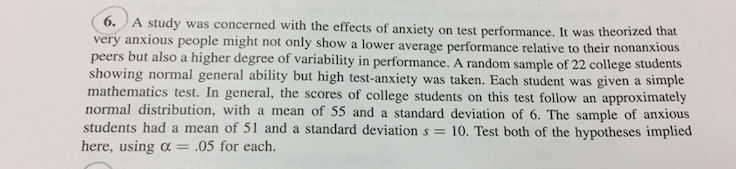# tudy was concerned with the effects of anxiety on test performance. It was theorized that very anxious people might not only show a lower average performance relati peers but als showing normal general ability but high test-anxiety was taken. Each student was given a simple mathematics test. In general, the scores of college students on this test follow an approximatey normal distribution, with a mean of 55 and a standard deviation of 6. The sample of anxious ve to their nonanxious o a higher degree of variability in performance. A random sample of 22 college students students had a mean of 51 and a standard deviation s 10. Test both of the hypotheses implied here, using α- 05 for each.

Question

Hi, not sure how to go about doing this hypothesis test. Would appreciate any help. thanks!help_outlineImage Transcriptionclosetudy was concerned with the effects of anxiety on test performance. It was theorized that very anxious people might not only show a lower average performance relati peers but als showing normal general ability but high test-anxiety was taken. Each student was given a simple mathematics test. In general, the scores of college students on this test follow an approximatey normal distribution, with a mean of 55 and a standard deviation of 6. The sample of anxious ve to their nonanxious o a higher degree of variability in performance. A random sample of 22 college students students had a mean of 51 and a standard deviation s 10. Test both of the hypotheses implied here, using α- 05 for each. fullscreen